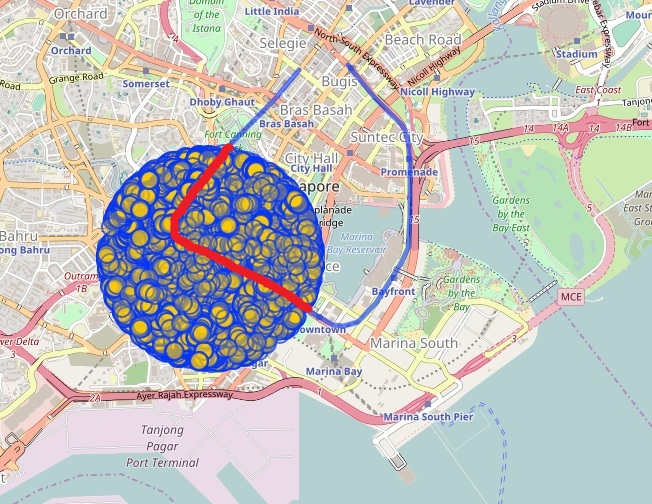# Find overlapping part about line and polygon

 1 I'm try to find Singapore MRT line in a range like below: ``````[out:json][timeout:100];(node(around:1000,1.284249, 103.844004)["name"~"Downtown Line MRT"];way(around:1000,1.284249, 103.844004)["name"~"Downtown Line MRT"];);out body;._;>;out skel qt; `````` (around:1000,1.284249, 103.844004) is a circle polygon and Downtown Line MRT is a line. How can I get the overlapping part only(red line in picture)? The query result will partially out of circle.asked 02 Jun '17, 03:37ob9619 26●2●2●4 accept rate: 0%

 0 You are indicating two issues - for a given poly-line and circle/polygon detect the poly-line's segments being inside the circle/polygon (clipping a poly-line by a circle/polygon). If you have not yet found these functions (answers) in the OSM public tools, here are some hints in bullets. Namely, the mentioned tools (should) have functions like - vectors to none crossing vectors transformation, point in (on, outside) a polygon, vectors to poly-lines transformation, change poly-line's orientation and some more, even simpler, functions and using them you (anyone) may create the missing functions. The simple/circle case's procedure. 1)From the poly-line edge-vector set detect/select the set {Vi} where any of the vectors Vi has a point inside the circle's bounding box. 2)If the line defined by a vector Vi crosses the circle, let us say in points A and B, select the vector Ej as part of Vi being inside the segment AB. The new set {Ej} contains only vectors being strictly inside the circle. 3)Connect the vectors from {Ej} into poly-line(s) and if necessary change the orientation of these. The general/polygon case's procedure is the same as the former (when "circle" is replaced by "polygon") except the step 2. Here, the procedure is more complex. The {Ej} set contains all Vi vectors having no common points with the polygon. If a vector Vi is crossing the polygon, let us say in a point X, then Vi should be divided by X into two new Ej vectors. Further, if a Vi is overlapping a polygon edge vector Pk then we replace Vi with its Ej parts being outside the vector Pk. Now, the set {Ej} contains vectors never crossing the polygon. You may prove that a vector Ej from this set {Ej} is strictly inside the polygon if, and only if, an inner point of it (e.g. the midpoint) is inside the polygon. Using the mentioned "point-in-polygon" function, from the set {Ej} we can keep only the elements being inside the polygon. Note that the former general/polygon model is (should be) used in a robust data preparation toll chain. For instance, we can detect and remove common area parts from one of the areas when two areas partly overlap (remove from a forest area part of it that is over a lake area), or find and recognize a missing section of a river area, or detect and remove anomalies caused by incorrect relations between river-lines (waterway=river) and the corresponding river-area objects and so on. For illustration of many similar examples see the river in the link https://goo.gl/p9lR3R . There are many (really large number) of virtual islands, wrong name positions, wrong river width, large redundancy, unnecessary rendering... just to mention some. These anomalies could be removed by using the mentioned general model. answered 09 Jun '17, 16:05sanser 695●38●39●55 accept rate: 5% Thank you for getting me back. In OSM, way is a set of nodes. Currently, I could find the nodes inside the circle polygon. However, sometimes there is only one point inside the circle polygon. How can I get the intersection points of line and circle? There should be two intersection points about one line and one circle which is not OSM nodes. I am still trying~ Do you have any idea? Thanks! (14 Jun '17, 03:26) ob9619
 toggle preview community wiki:

By Email:

Markdown Basics

• *italic* or _italic_
• **bold** or __bold__
• image?![alt text](/path/img.jpg "title")
• numbered list: 1. Foo 2. Bar
• to add a line break simply add two spaces to where you would like the new line to be.
• basic HTML tags are also supported

Question tags:

×89
×64
×30
×1

question asked: 02 Jun '17, 03:37

question was seen: 3,296 times

last updated: 14 Jun '17, 03:28